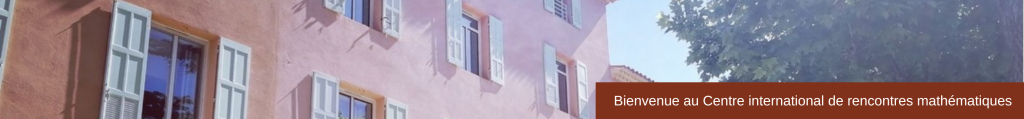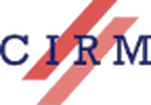CONFERENCE
AGCT-15: Arithmetics, Geometry, Cryptography and Coding Theory
May 18 – 22, 2015
 This conference deals with the interactions between arithmetics, algebraic geometry and computer sciences, in particular coding theory and cryptology. More precisely, the research themes of the conference are: – Number theory, asymptotics of global fields– Arithmetic Geometry, algebraic curves over finite fields or number fields, abelian varieties. Theoretical and algorithmic aspects of point counting– Coding theory, algebraic and algebraic geometry codes, codes from curves and higher dimensional varieties, decoding algorithms– Boolean functions, bent and APN functions, constructions of families of bent and hyperbent functions. Scientific and Organizing Committee Alp Bassa (Bogazici University)Alain Couvreur (Ecole polytechnique)David Kohel (Aix-Marseille Université)
Speakers
 Nurdagül Anbar (Technical University of Denmark) On idempotent quadratic functions and the weight distribution of subcodes of Reed-Muller codes Christine Bachoc (Université de Bordeaux) An Analogue of Vosper’s Theorem for Extension Fields Régis Blache (Université des Antilles et de la Guyane) Valuations of Exponential Sums, Congruences for L-Functions, andConsequences Irène Bow (University of Ulm) Computing  \$L\$-functions of superelliptic curves Alain Couvreur (Ecole polytechnique) An upper bound on the number of points of a projective variety and a proof of a conjecture of Ghorpade and Lachaud Agnès David (Université de Franche-Comté) Of Kisin varieties and Galois deformation rings Florent Demeslay (Université de Caen) Class Formula in Positive Characteristic Ernst-Ulrich Gekeler (University of Saarland) Algebraic Curves with Many Rational Points over Non-Prime Finite Fields Sudhir Ghorpade (Indian Institute of Technology Bombay) Tsfasman-Boguslavsky Conjecture for Polynomial Systemsand Generalized Hamming Weights of Projective Reed-Muller Codes Aurore Guillevic (Inria Saclay) Computing Discrete logarithms in GF(p n): Practical Improvement of the Individual Logarithm Step Emmanuel Hallouin (Université Jean Jaurès Toulouse) Weil Bounds of Higher Orders Masaaki Homma (Kanagawa University) An Analogue of a Theorem of Tallini on Plane Curves over Finite Fields Everett Howe (Center for Communications Research, San Diego) Polarizations on E x E and genus-4 curves with many points Annamaria Iezzi (Aix-Marseille Université) Towards maximal singular curves over finite fields Antoine Joux (Laboratoire d’informatique de Paris 6) A Simplified Setting for Discrete Logarithms in Small Characteristic Finite Fields Grigory Kabatiansky (Russian Academy of Sciences) On double sparse compressed sensing via coding theory and its different applications Kamal Khuri-Makdisi (American University of Beirut) Bounding Brill-Noether loci over finite fields, with applications to Jacobian arithmetic David Kohel (Aix-Marseille Université) On extremal traces and RM in genus 2 Gilles Lachaud (Aix-Marseille Université) On the Number of Points of Algebraic Sets  over Finite Fields Christine Lauter (Microsoft Research Redmond) A new hard problem?  (Ring) Learning With Errors Reynald Lercier (Université Rennes 1) Covariant Algebra of the Binary Nonic and the Binary Decimic Bernard Le Stum (Université Rennes 1) An Introduction to Rigid Cohomology Christine Lauter (Microsoft Research Redmond) A new hard problem?  (Ring) Learning With Errors Reynald Lercier (Université Rennes 1) Covariant Algebra of the Binary Nonic and the Binary Decimic Bernard Le Stum (Université Rennes 1) An Introduction to Rigid Cohomology Irene Marquez Corbella (Inria Rocquencourt) Structural Cryptanalysis of McEliece Schemes with Algebraic Geometry Codes Guillermo Matera (UNGS and CONICET) Estimates on the Number of Rational Points of Singular Complete Intersections over a Finite Field and Applications Tohru Nakashima (Kyoto University) AG Codes from Restriction of Vector Bundles to Divisors Nhut Nguyen (Technical University of Denmark) Some developments on towers over cubic finite fields Johan Nielsen (INRIA Saclay) Power Decoding of Hermitian One-Point Codes in Sub-Quadratic Time Ekin Ozman (Bogazici University) Bad Reduction of Genus Three Curves with Complex Multiplication Marc Perret (Université Jean Jaurès Toulouse) Graph based strategy to exhibit good recursive towers Alena Pirutka (CNRS & École polytechnique) Algebraic cycles on varieties over finite fields Christophe Ritzenthaler (Université Rennes 1) A new proof of a Thomae like-formula for non hyperelliptic genus 3 curves Yieh-Dar Shieh (Aix-Marseille Université) Character theory and Sato-Tate groups Jeroen Sijsling (University of Warwick) Branches and Descent Benjamin Smith (INRIA / École polytechnique) Slightly more practical quantum factoring through number theory Peter Stevenhagen (University of Leiden) Adelic points of elliptic curves Claudio Stirpe (IIS Marconi, Anagni) New results on class numberone problem for function fields Marco Streng (University of Leiden) Generators of the group of modularunits Andrew Sutherland (MIT) Computing the image of Galois representations attached to elliptic curves Seher Tutdere (Gebze Technical University) On the Torsion-Limit for Algebra Function Fields Florent Ulpat-Rovetta (Aix-Marseille Université) Construction of genus two curve starting from their invariants in characteristic 5 Vanessa Vitse (Université Grenoble 1) Field extensions and index calculus on algebraic curve Serge Vladuts (Aix-Marseille Université) Locally recoverable codes on algebraic curves